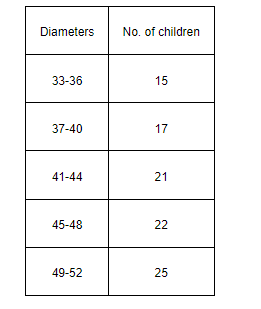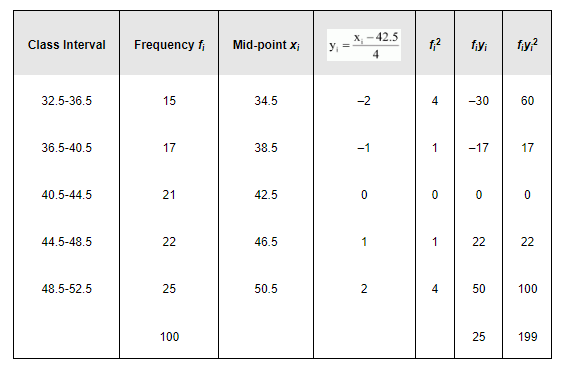Deepak Scored 45->99%ile with Bounce Back Crack Course. You can do it too!

# The diameters of circles (in mm) drawn in a design are given below:

Question:

The diameters of circles (in mm) drawn in a design are given below:Solution:Here, N = 100, h = 4

Let the assumed mean, A, be 42.5.

Mean, $\bar{x}=A+\frac{\sum_{i=1}^{5} f_{i} y_{i}}{N} \times h=42.5+\frac{25}{100} \times 4=43.5$

$\operatorname{Variance}\left(\sigma^{2}\right)=\frac{h^{2}}{N^{2}}\left[N \sum_{i=1}^{3} f_{i} y_{i}^{2}-\left(\sum_{i=1}^{3} f_{i} y_{i}\right)^{2}\right]$

$=\frac{16}{10000}\left[100 \times 199-(25)^{2}\right]$

$=\frac{16}{10000}[19900-625]$

$=\frac{16}{10000} \times 19275$

$=30.84$

$\therefore$ Stan dard deviation $(\sigma)=5.55$